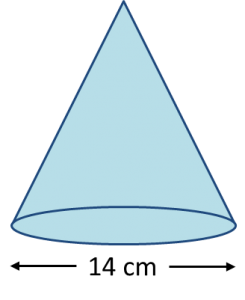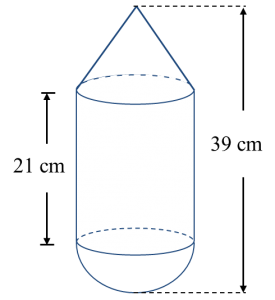# 6.5.1 Volume of Three Dimensional Shapes, PT3 Practice

 
 
6.5.1 Volume of Three Dimensional Shapes, PT3 Practice
 

  Question 1:
 
The diagram below shows a cone with diameter 14 cm and height 6 cm.Find the volume of the cone, in cm3.
 

 
Solution:
    $\begin{array}{l}\text{Volume of a cone}\\ =\frac{1}{3}\pi {r}^{2}h\\ =\frac{1}{\overline{)3}}×\frac{22}{\overline{)7}}×{7}^{\overline{)2}}×\overline{)6}2\\ =308{\text{cm}}^{3}\end{array}$

 

 
 
 
 
Question 2:
 In the pyramid shown, the base is a rectangle.If the volume is 20 cm2, find the height of the pyramid, in cm.
 
 

  Solution:
 
  $\begin{array}{l}\text{Volume of a pyramid}=\frac{1}{3}×\text{base area}×h\\ \frac{1}{3}×\text{base area}×h=20\\ \frac{1}{3}×5×4×h=20\\ \text{}\frac{20}{3}×h=20\\ \text{}h=\overline{)20}×\frac{3}{\overline{)20}}\\ \text{}h=3\text{cm}\end{array}$

 
 
 
 
 
Question 3:
 
Diagram below shows a composite solid consisting of a right circular cone, a right circular cylinder and a hemisphere.The volume of the cylinder is 1650 cm3. Calculate the height, in cm, of the cone.
 
   
 
 

  Solution:
 
  $\begin{array}{l}\text{Volume of a cylinder}=\pi {r}^{2}h\\ \frac{22}{7}×{r}^{2}×21=1650\\ \text{}{r}^{2}=\frac{1650×7}{22×21}\\ \text{}=25\\ \text{}r=5cm\\ \text{Thus the height of the cone}\\ =39-5-21\\ =13cm\end{array}$